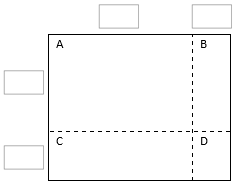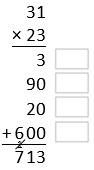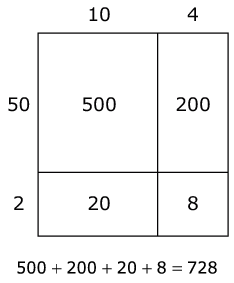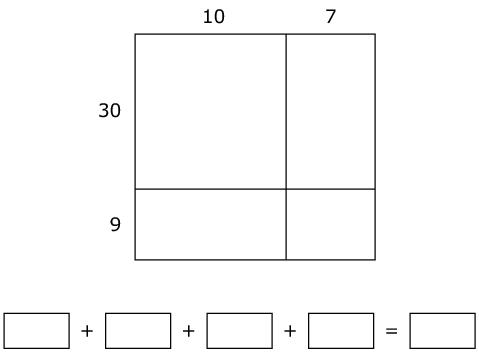# Multi-Digit Multiplication

## Objective

Multiply two-digit by two-digit numbers using four partial products.

## Common Core Standards

### Core Standards

?

• 4.NBT.B.5 — Multiply a whole number of up to four digits by a one-digit whole number, and multiply two two-digit numbers, using strategies based on place value and the properties of operations. Illustrate and explain the calculation by using equations, rectangular arrays, and/or area models.

?

• 3.NBT.A.3

• 4.NBT.A.1

• 4.NBT.B.4

• 3.OA.B.5

• 3.OA.C.7

## Criteria for Success

?

1. Understand that products can be computed by decomposing numbers into base-ten units, finding partial products of these base-ten units, then adding these partial products together based on the distributive property (e.g., 36 x 54 = (30 x 50) + (30 x 4) + (6 x 50) + (6 x 4)). (Note that students need not know the term “distributive property.”)
2. Multiply a two-digit whole number by a two-digit whole number using area models and the partial products algorithm.
3. Estimate products by rounding factors to the largest place value.
4. Solve one-step word problems involving multiplication of two-digit by two-digit numbers (on the Problem Set and Homework) (MP.4).

## Tips for Teachers

?

• Throughout the remainder of the topic, the main visual model used is the area model. If students seem to be struggling with place value understanding or don’t yet seem ready for the area model for some other reason, you might create a lesson to use before this one that focuses on the use of the base ten block array and/or graph paper array to build understanding toward the area model. The applet Partial Product Finder by The Math Learning Center may be helpful if you take that route: . (See Unit-Specific Intellectual Prep section of the Unit Overview for examples of those representations.)
• Lessons 18 through 20 move through the various representations much more quickly than was done in Topic C, since they are already familiar with these representations and just extending them to two-digit by two-digit multiplication. If students struggled in Topic C, you might choose to use a flex day to solidify the strategies with two-, three-, and four-digit by one-digit multiplication or to extend Topic D over more days, having it more closely resemble Topic C.

#### Remote Learning Guidance

If you need to adapt or shorten this lesson for remote learning, we suggest prioritizing Anchor Task 2 (benefits from worked example). Find more guidance on adapting our math curriculum for remote learning here.

#### Fishtank Plus

• Problem Set
• Student Handout Editor
• Vocabulary Package

?

### Problem 1

Mr. Wynn now wants to cover a part of the gym with butcher block paper to work on a large painting. He covers a section of the gym that is 30 feet long and 35 feet wide. Then he realizes he needs a bit more space for the painting and adds a section of butcher block that is 4 feet long and 35 feet wide.

a. How many square feet of butcher block paper did Mr. Wynn use for his painting?

b. What is the total length and width of Mr. Wynn’s painting?

#### References

EngageNY Mathematics Grade 4 Mathematics > Module 3 > Topic H > Lesson 36Concept Development

Grade 4 Mathematics > Module 3 > Topic H > Lesson 36 of the New York State Common Core Mathematics Curriculum from EngageNY and Great Minds. © 2015 Great Minds. Licensed by EngageNY of the New York State Education Department under the CC BY-NC-SA 3.0 US license. Accessed Dec. 2, 2016, 5:15 p.m..

Modified by The Match Foundation, Inc.

### Problem 2

Label the area model to represent 31 x 23 and to find that product.

a. Decompose each number into its base-ten units (tens and ones) and write them in the boxes on each side of the rectangle.b. Label regions A, B, C, and D with their areas. Show your reasoning.

c. Find the product that the area model represents. Show your reasoning.

Here is one way to calculate 31 x 23. Each number with a box gives the area of one region in the area model.a. In the boxes next to each number, write the letter of the corresponding region.

b. There is a 1 above the hundreds digit in the sum. What does that 1 represent?

#### References

EngageNY Mathematics Grade 4 Mathematics > Module 3 > Topic H > Lesson 36Concept Development

Grade 4 Mathematics > Module 3 > Topic H > Lesson 36 of the New York State Common Core Mathematics Curriculum from EngageNY and Great Minds. © 2015 Great Minds. Licensed by EngageNY of the New York State Education Department under the CC BY-NC-SA 3.0 US license. Accessed Dec. 2, 2016, 5:15 p.m..

Modified by The Match Foundation, Inc.
Open Up Resources Grade 6 Unit 5 Lesson 7 (Teacher Version)Activity 4

Modified by The Match Foundation, Inc.

### Problem 3

Estimate the product. Then use any method to solve.

a. 46 x 37

b. 81 x 52

c. 68 x 73

#### References

EngageNY Mathematics Grade 4 Mathematics > Module 3 > Topic H > Lesson 36Concept Development

Grade 4 Mathematics > Module 3 > Topic H > Lesson 36 of the New York State Common Core Mathematics Curriculum from EngageNY and Great Minds. © 2015 Great Minds. Licensed by EngageNY of the New York State Education Department under the CC BY-NC-SA 3.0 US license. Accessed Dec. 2, 2016, 5:15 p.m..

Modified by The Match Foundation, Inc.

## Problem Set & Homework

#### Discussion of Problem Set

• Why is it possible to represent #8 with an area model even though it is not an area problem?
• When working with a two-digit by two-digit multiplication problem, how many partial products are there usually? What if one of the two-digit numbers is a multiple of ten (i.e., ends in zero)? What if they both are?
• How did our previous work with area models and partial products help us to be ready to solve two-digit by two-digit multiplication problems using four partial products?
• How could you explain to someone that ones x tens equals tens but tens x tens equals hundreds

?

Brittany solves 52 x 14 using an area model.Use the same reasoning as Brittany to solve 39 x 17. Find each partial product in the area model and then fill in the blanks to complete the equation.#### References

Smarter Balanced Assessment Consortium: Sample Items Sample Item #3443

Sample Item #3443 from Smarter Balanced Assessments' Sample Items is made available by Smarter Balanced Assessment Consortium. © The Regents of the University of California – Smarter Balanced Assessment Consortium. Accessed Jan. 8, 2018, 2:21 p.m..

### Mastery Response

?